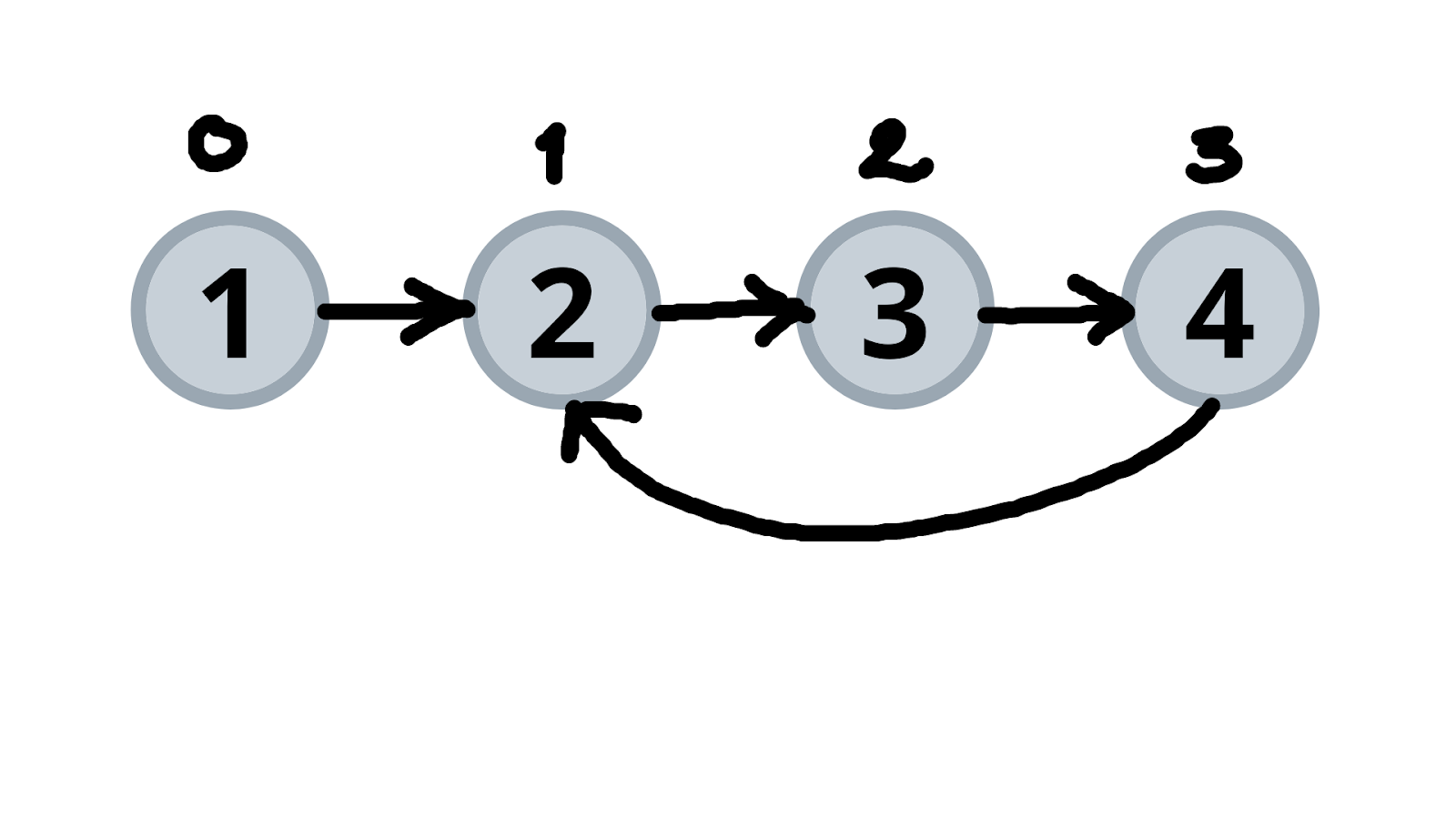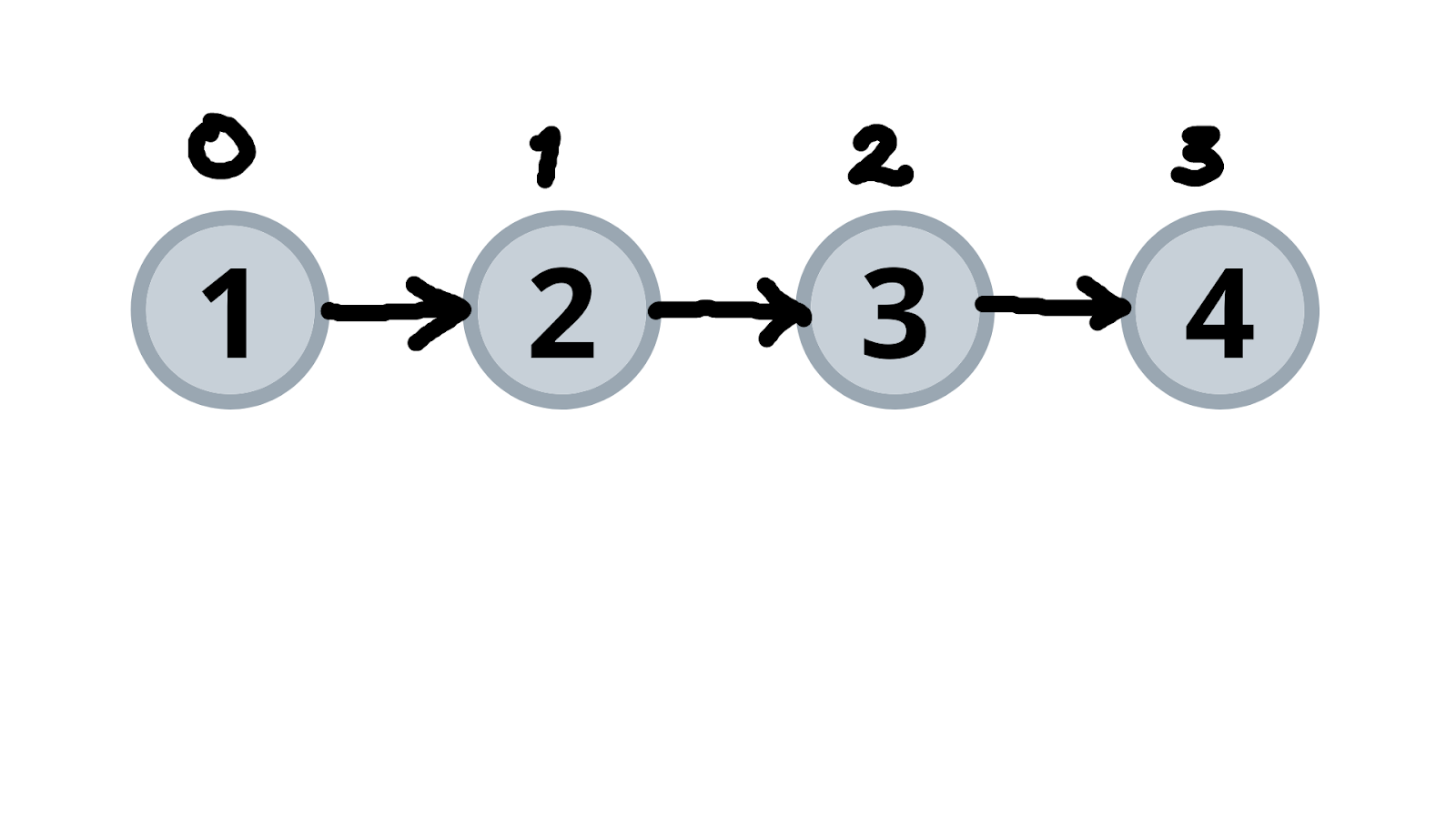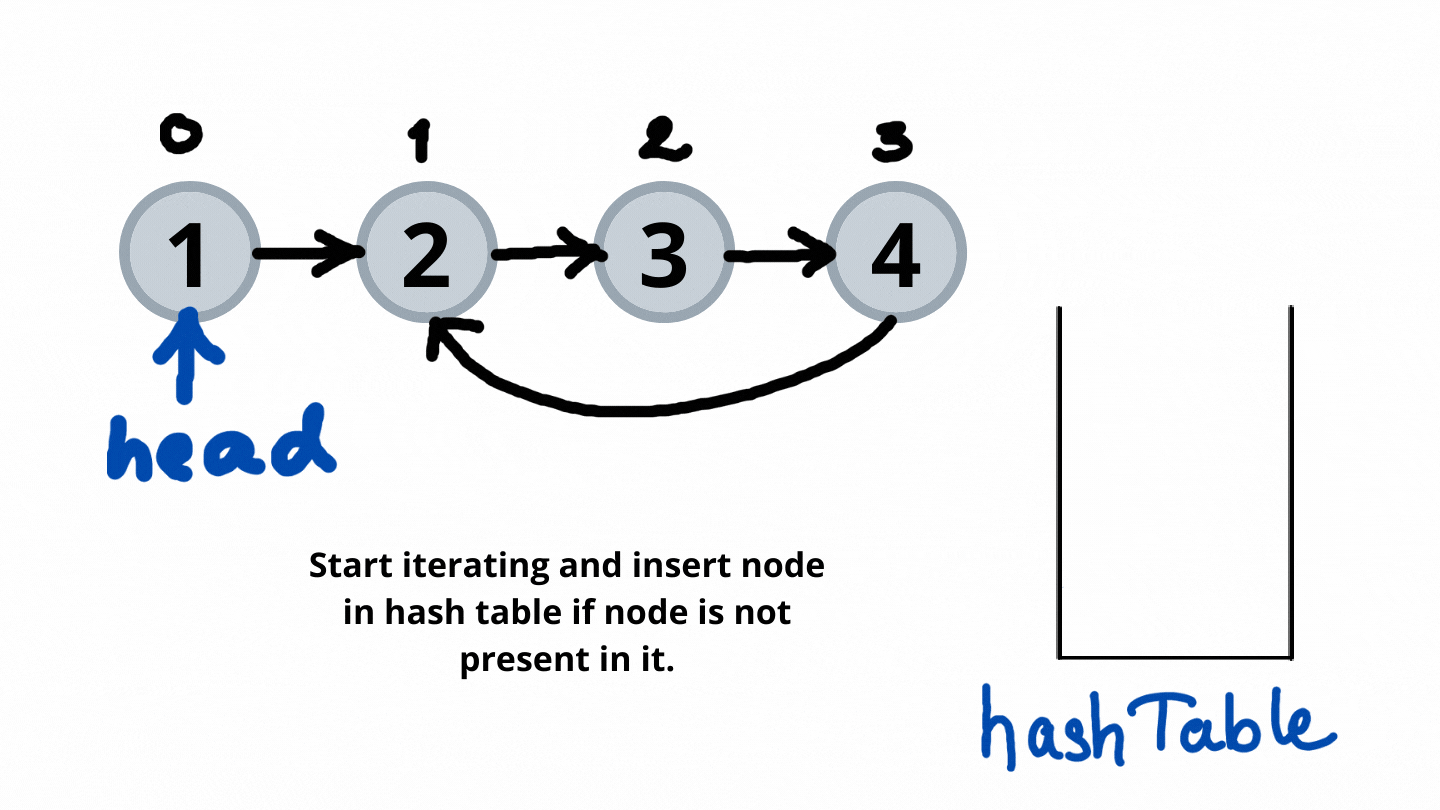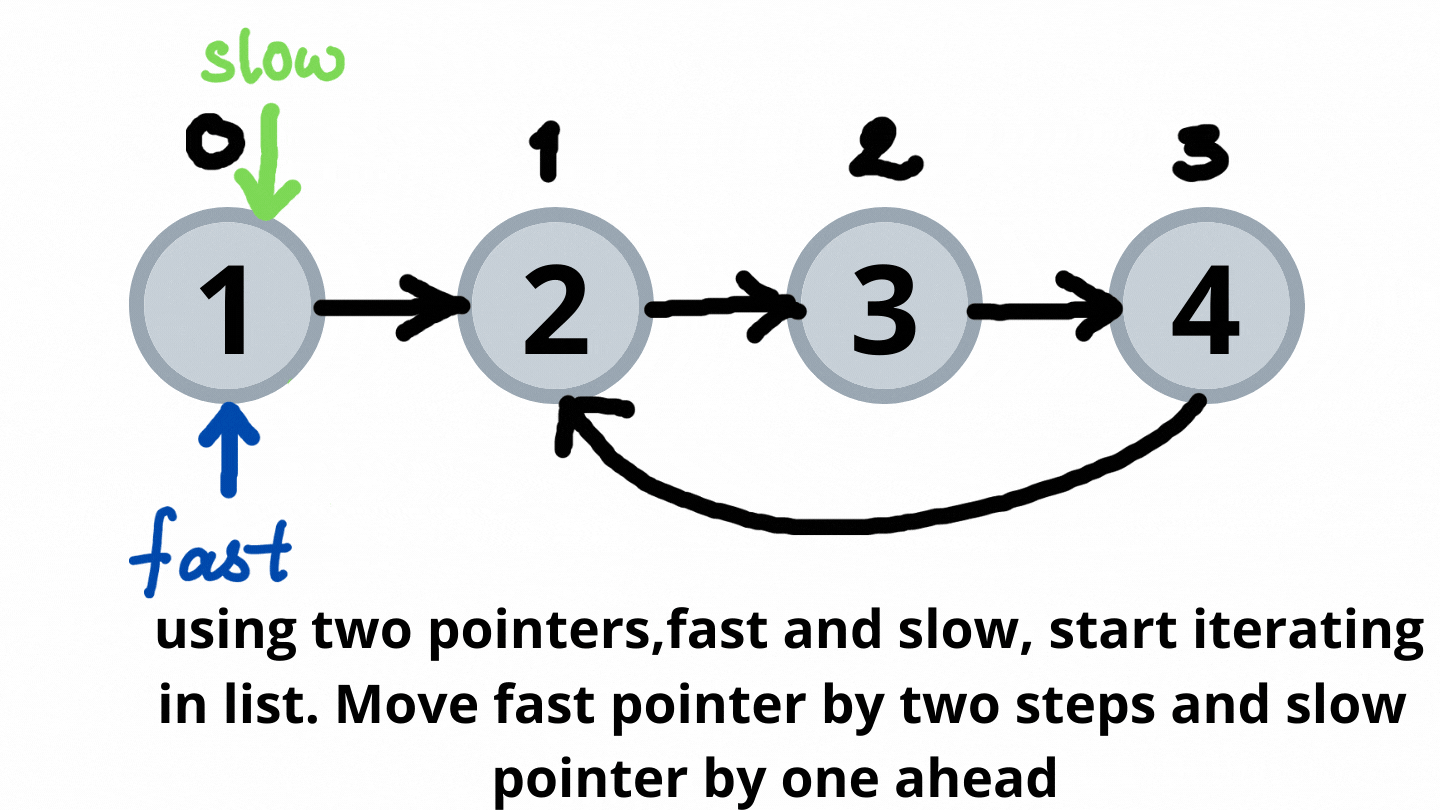# Detect a Cycle in a Linked List

In this article, we will solve the most asked interview question: Detect a Cycle in a Linked List

Problem Statement: Given head, the head of a linked list, determine if the linked list has a cycle in it. There is a cycle in a linked list if there is some node in the list that can be reached again by continuously following the next pointer.

Return true if there is a cycle in the linked list. Otherwise, return false.

Examples:

```Example 1:
Input:
Output:
true
Explanation: Here, we can see that we can reach node at position 1 again by following the next pointer. Thus, we return true for this case.```
```Example 2:
Input:
Output:
false
Explanation: Neither of the nodes present in the given list can be visited again by following the next pointer. Hence, no loop exists. Thus, we return false.```

Solution: Hashing

Approach:

We need to keep track of all the nodes we have visited till now so that once we visit the same node again we can say that a cycle is detected. The process is as follows:

• Use a hash table for storing nodes.
• Start iterating through the lists.
• If the current node is present in the hash table already, this indicates the cycle is present in the linked list and returns true.
• Else move insert the node in the hash table and move ahead.
• If we reach the end of the list, which is NULL, then we can say that the given list does not have a cycle in it and hence we return false.

Dry Run:Code:

## C++ Code

``````#include<bits/stdc++.h>
using namespace std;

class node {
public:
int num;
node* next;
node(int val) {
num = val;
next = NULL;
}
};

//utility function to insert node in the list
void insertNode(node* &head,int val) {
node* newNode = new node(val);

if(head == NULL) {
return;
}

node* temp = head;
while(temp->next != NULL) temp = temp->next;

temp->next = newNode;
return;
}

//utility function to create cycle
void createCycle(node* &head,int a,int b) {
int cnta = 0,cntb = 0;
node* p1 = head;
node* p2 = head;
while(cnta != a || cntb != b) {
if(cnta != a) p1 = p1->next, ++cnta;
if(cntb != b) p2 = p2->next, ++cntb;
}
p2->next = p1;
}

//utility function to detect cycle
bool cycleDetect(node* head) {
unordered_set<node*> hashTable;
while(head != NULL) {
if(hashTable.find(head) != hashTable.end()) return true;
}
return false;
}

int main() {
node* head = NULL;
createCycle(head,1,3);//creating cycle in the list
cout<<"Cycle detected\n";
else
cout<<"Cycle not detected\n";
return 0;
}
``````

Output: Cycle detected

Time Complexity: O(N)

Reason: Entire list is iterated once.

Space Complexity: O(N)

Reason: All nodes present in the list are stored in a hash table.

## Java Code

``````import java.util.*;
class Node {
int num;
Node next;
Node(int val) {
num = val;
next = null;
}
}

//utility function to insert node in the list
class TUF{
static Node insertNode(Node head,int val) {
Node newNode = new Node(val);

if(head == null) {
}

Node temp = head;
while(temp.next != null) temp = temp.next;

temp.next = newNode;
}

//utility function to create cycle
static void createCycle(Node head,int a,int b) {
int cnta = 0,cntb = 0;
Node p1 = head;
Node p2 = head;
while(cnta != a || cntb != b) {
if(cnta != a)
{p1 = p1.next; ++cnta;}
if(cntb != b)
{p2 = p2.next; ++cntb;}
}
p2.next = p1;
}

//utility function to detect cycle
static boolean cycleDetect(Node head) {
HashSet <Node> hashTable=new HashSet<>();
while(head != null) {
}
return false;
}

public static void main(String args[]) {
Node head = null;
createCycle(head,1,3);//creating cycle in the list
System.out.println("Cycle detected");
else
System.out.println("Cycle not detected");

}
}``````

Output: Cycle detected

Time Complexity: O(N)

Reason: Entire list is iterated once.

Space Complexity: O(N)

Reason: All nodes present in the list are stored in a hash table.

Solution: Slow and Faster Pointer

Approach:

We will use two pointers with different steps forward. The process is as follows:-

• We will take two pointers, namely fast and slow. Fast pointer takes 2 steps ahead and slow pointer takes 2 points ahead.
• Iterate through the list until the fast pointer is equal to NULL. This is because NULL indicates that there is no cycle present in the given list.
• Cycle can be detected when fast and slow pointers collide.

Dry Run:Code:

## C++ Code

``````#include<bits/stdc++.h>
using namespace std;

class node {
public:
int num;
node* next;
node(int val) {
num = val;
next = NULL;
}
};

//utility function to insert node in the list
void insertNode(node* &head,int val) {
node* newNode = new node(val);

if(head == NULL) {
return;
}

node* temp = head;
while(temp->next != NULL) temp = temp->next;

temp->next = newNode;
return;
}

//utility function to create cycle
void createCycle(node* &head,int a,int b) {
int cnta = 0,cntb = 0;
node* p1 = head;
node* p2 = head;
while(cnta != a || cntb != b) {
if(cnta != a) p1 = p1->next, ++cnta;
if(cntb != b) p2 = p2->next, ++cntb;
}
p2->next = p1;
}

//utility function to detect cycle
bool cycleDetect(node* head) {
if(head == NULL) return false;
node* fast = head;
node* slow = head;

while(fast->next != NULL && fast->next->next != NULL) {
fast = fast->next->next;
slow = slow->next;
if(fast == slow) return true;
}
return false;
}

int main() {
node* head = NULL;
createCycle(head,1,3);//creating cycle in the list
cout<<"Cycle detected\n";
else
cout<<"Cycle not detected\n";
return 0;
}
``````

Output: Cycle detected

Time Complexity: O(N)

Reason: In the worst case, all the nodes of the list are visited.

Space Complexity: O(1)

Reason: No extra data structure is used.

## Java Code

``````import java.util.*;
class Node {
int num;
Node next;
Node(int val) {
num = val;
next = null;
}
}

//utility function to insert node in the list
class TUF{
static Node insertNode(Node head,int val) {
Node newNode = new Node(val);

if(head == null) {
}

Node temp = head;
while(temp.next != null) temp = temp.next;

temp.next = newNode;
}

//utility function to create cycle
static void createCycle(Node head,int a,int b) {
int cnta = 0,cntb = 0;
Node p1 = head;
Node p2 = head;
while(cnta != a || cntb != b) {
if(cnta != a)
{p1 = p1.next; ++cnta;}
if(cntb != b)
{p2 = p2.next; ++cntb;}
}
p2.next = p1;
}

//utility function to detect cycle
static boolean cycleDetect(Node head) {
if(head == null) return false;
Node fast = head;
Node slow = head;

while(fast.next != null && fast.next.next != null) {
fast = fast.next.next;
slow = slow.next;
if(fast == slow) return true;
}
return false;
}

public static void main(String args[]) {
Node head = null;
createCycle(head,1,3);//creating cycle in the list
System.out.println("Cycle detected");
else
System.out.println("Cycle not detected");

}
}``````

Output: Cycle detected

Time Complexity: O(N)

Reason: In the worst case, all the nodes of the list are visited.

Space Complexity: O(1)

Reason: No extra data structure is used.

Special thanks to Dewanshi Paul for contributing to this article on takeUforward. If you also wish to share your knowledge with the takeUforward fam, please check out this article